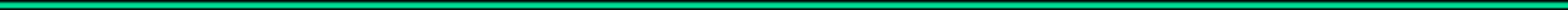Home News What is baudline? Screenshots Download FAQ Manual Search Solutions Mystery Signal Contact
equalization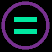This window models the channel shape and corrects the global frequency response by applying an inverse curve.  This is known as "equalizing the channel" which is quite similar to how an audio equalizer might be used to correct  the frequency response of the room / speaker combination in a home theater or recording studio environment.  Note that this equalizer works only in the frequency domain; it does not correct for multipath, so it is not yet a true equalizer in the modem sense.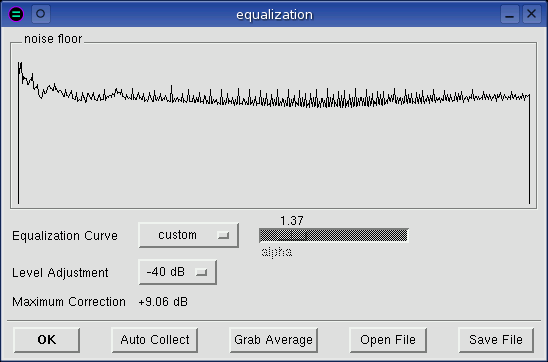noise floor Noise floor is a pictorial representation of the channel shape.  The inverse of this frequency response is the equalization curve.  Like the Average or Spectrum windows, this is a frequency vs dB display.Equalization Curve Choose a preset equalization curve, build a custom curve, or turn off equalization with the flat setting.  Note that custom equalization curves are automatically saved on program exit and loaded next time baudline is started. alpha This slider is only sensitive when the 1 / f ^ alpha equalization curve is set.  Alpha represents the slope in -dB / octave.  Note that alpha=1.0 is proper 1 / f noise, and alpha=3.0 is pink noise. Level Adjustment Since the goal of equalization is to make the frequency response as flat as possible, noise floors that have strong tones will result in large corrections.  This will push the spectral energy up which could result in clamping at the 0 dB level and it might impair visualization resolution or scaling.  This control reduces the post equalization spectral levels so that strongly equalized signals can be brought back into a usable zone.  Maximum Correction This displays in dB the maximum value of the current equalization curve. Auto Collect There are two modes of operation -  record and pause.  In record mode, the custom curve is cleared, average collection begins, and the name of the button changes to "Stop Collect."  The noise floor displays the equalization curve as it collects.  The longer duration the spectrum is collected, the smoother it gets, and the more accurately it represents the true channel shape.  Hitting the Stop Collect button halts the average spectra collection, inverts the curve, and applies it as the new custom curve.  In pause mode, the custom curve is cleared, the selected Spectrogram data (or ALL data if none) is average collected, and the frequency response is applied as the new custom curve.  Note that both of these modes of operation are equivalent to manually collecting data in the average window and then hitting the Grab Average button which is described below.  Grab Average Copy the current Average destination spectrum and paste it here as the custom equalization curve.  Open/Save File Load a new custom equalization curve from a file.  Or save the currently selected equalization curve to a specific file.  The file format is the same as is used in the average window.  The format is two column (Hz, dB) ASCII text which can be plotted with xgraph or gnuplot.

progress barThis window pops up every time a lengthy calculation is being performed.  It displays the current status from 0% to 100% and it has a Stop button for halting the current calculation.  This window can pop up from a paste done in the average or histogram windows, from an Auto Collect operation done in the equalization window, or from a stdout paste operation.

transform sizeThis selects the size of the Fast Fourier Transform (FFT) that is used internally.  Transform sizes range from 128 up to 65536 bins in power of 2 increments.  There are benefits to using both larger and smaller FFT sizes.  Larger FFT's have a finer frequency resolution and a higher SNR (this means they are better at extracting weak signals).  Smaller FFT's use less CPU resources and they have a finer time resolution (they blur time variant signals less).  Note that the number of frequency bins is the value of half of the transform size.  So a 2048 point transform generates 1024 pixels width of frequency resolution.  If the spectral windows are resized smaller than this value, the "extra" information is just discarded not rescaled unless a Hz zoom factor is selected. The bin resolution (Hz / bin) which is how many Hz are in each FFT bin can be found by looking in the popdown options section of the Drift Integrator window.  The bin resolution is a function of both transform size and sample rate.

windowing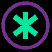The windowing function is applied by multiplication to a signal slice before the Fourier transform is done.  Windowing is important because it reduces wraparound leakage.  It widens the lobe width while it increases the time resolution detail.Note that the Fourier and Correlation transforms use the windowing functions while the Raster and Histogram transforms ignore it.

Windowing function characteristics can vary dramatically.  Some windows are designed for general purpose work while others are very specialized.  Several of the windows even have an adjustable beta variable that can modify the windowing shape.

The Hanning, Blackman (default), and Kaiser (beta=14) windows are good for general spectral analysis work.  The Welch and Gaussian windows have special feature extraction abilities that are optimized respectively for weak signal and short duration (enhanced time-domain resolution) uses.  See the windowing gallery below for details and notes about each specific window: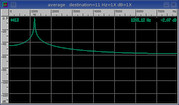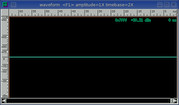Square
 Formula: 1. Overlap: 50% SINAD: 57.6 dB Notes: Flat top.  The square window has the narrowest lobe width at top center but it also has a lot of wrap leakage and wide skirts.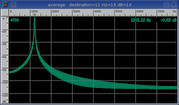Parzen
 Formula: 1. - abs(x / N - 1.) Overlap: 29.5% SINAD: 88.9 dB Notes: Triangle shape.  Oscillating side bands.  Also known as the Bartlett window.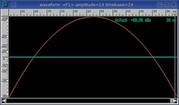Welch
 Formula: 1. - (x / N - 1.)^2 Overlap: 34.8% SINAD: 82.8 dB Notes: Parabolic shape.  Maximum sensitivity for weak signal work.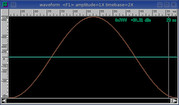Hanning
 Formula: 0.5 - 0.5 * cos(pi * x / N) Overlap: 26.6% SINAD: 96.6 dB Notes: Cosine shape.  Good for general purpose use.  Also known as the von Hann window.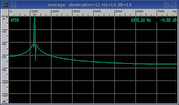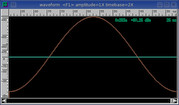Hamming
 Formula: 0.54 - 0.46 * cos(pi * x / N) Overlap: 28.7% SINAD: 35.1 dB Notes: Raised cosine shape introduces minor leakage.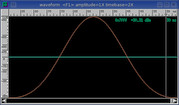Blackman
 Formula: 0.42 - 0.5 * cos(pi * x / N) + 0.08 * cos(2 * pi * x / N) Overlap: 22.5% SINAD: 95.9 dB Notes: Default.  Thinner version of Hanning window.  Good for general purpose use.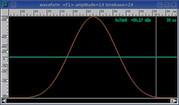Kaiser
 Formula: bessel(beta * sqrt(1. - (1. - x / N)^2)) / bessel(beta) Beta: 14. Overlap: 17.8% SINAD: 95.6 dB Notes: A beta of 14 is good for general purpose use.  Thinner and adjustable beta version of Blackman window.  Increase beta for enhanced time-domain resolution.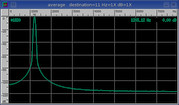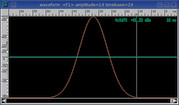Gaussian
 Formula: e^(-0.5 * (beta * (1. - x * N))^2) Beta: 6. Overlap: 11.3% SINAD: 96.0 dB Notes: Thinner version of Kaiser window.  Increased beta range for enhanced time-domain resolution.  Pull out the individual symbols of an FSK modem at the highest beta settings.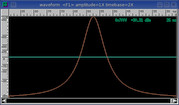Cauchy
 Formula: 1. / (1. + (beta * (1. - x * N))^2) Beta: 6. Overlap: 14.3% SINAD: 71.0 dB Notes: Slightly rounded exponential.  Increased beta range for enhanced time-domain resolution.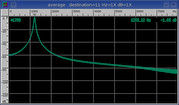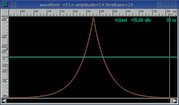Poisson
 Formula: e^(beta * (x / N - 1.)) Beta: 6. Overlap: 11.5% SINAD: 81.4 dB Notes: Pointy exponential.  Increased beta range for enhanced time-domain resolution.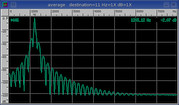Tukey
 Formula: 0.5 - 0.5 * cos(pi / beta * x / N) Beta: 6. Overlap: 46.3% SINAD: 26.3 dB Notes: Flat top with cosine tapered edges.  Identical to Hanning window at max beta.

The overlap percent was calculated using the 100% Optimum Slide Size value from the Drift Integrator window.

The SINAD measurement was made with a 0 dB digital amplitude 1209.10 Hz sine wave.  Note that SINAD is basically equivalent to SNR for these sine windowing test measurements.